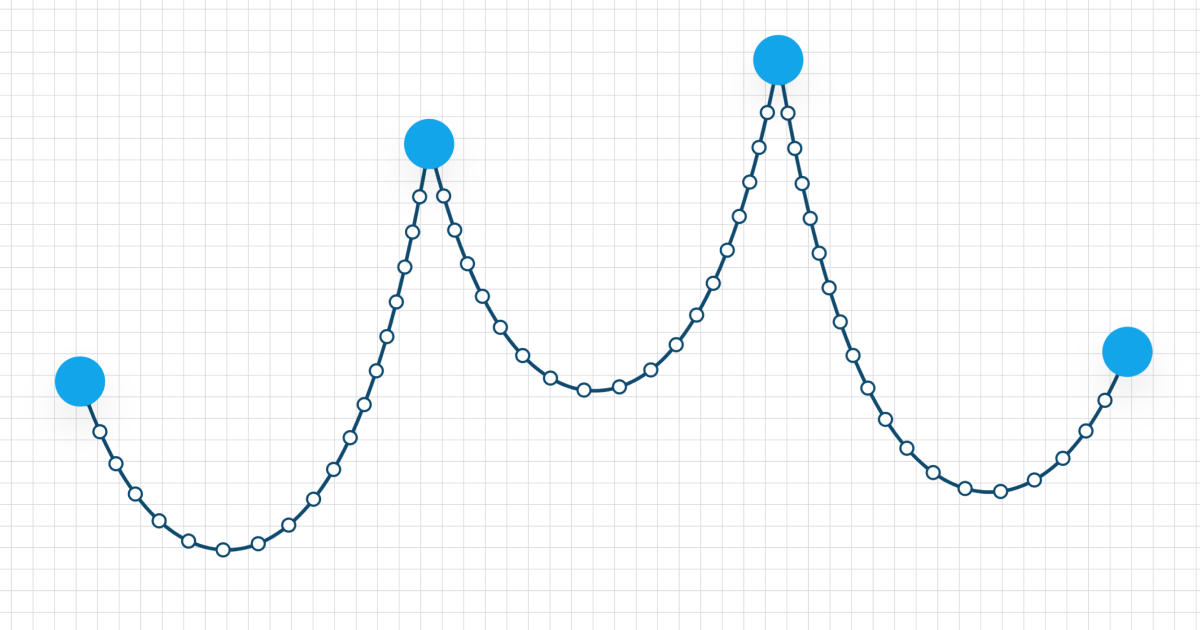# catenary-curve2.0.1 • Public • Published

# Catenary Curve - Approximates the catenary curve between two pointsOne would assume it's easy to draw a hanging rope or chain between two points, but it's not, especially if you want it to look realistic.

This TypeScript/JavaScript library provides a function that, given two points and a chain length, returns an approximation of the catenary curve as quadratic curve points.

It also provides helper methods to draw the result to a 2D canvas.

## How to use

`npm install --save catenary-curve`
```import { getCatenaryCurve, drawResult, Point } from 'catenary-curve'

const p1: Point = { x: 200, y: 300 }
const p2: Point = { x: 250, y: 400 }

const context = canvas.getContext('2d')

context.beginPath()
context.lineWidth = 1
context.strokeStyle = 'black'

const result = getCatenaryCurve(p1, p2, 500)
drawResult(result, context)

context.stroke()```

## Exports

### getCatenaryCurve

```function getCatenaryCurve(
point1: Point,
point2: Point,
chainLength: number,
options?: CatenaryOptions
): CatenaryCurveResult```

Provide the start and end point and the length of the chain. It returns an object with the approximated values.

```{
"start": [
480.3333333333333,
213.5920866272993
],
"curves": [
[
485.2094017094017,
237.1602015730591,
490.0854700854701,
258.6001522901396
],
[
846.0384615384615,
202.65311976627885,
850.9145299145299,
175.99552022287116
],
[
855.7905982905983,
149.33792067946348,
860.6666666666666,
120.05645761711651
]
]
}```

This resulting object can be passed to the `drawResult` method:

```const result = getCatenaryCurve(p1, p2, 500)
drawResult(result, context)```

Alternatively you can also do the drawing yourself:

```const result = getCatenaryCurve(p1, p2, 500)

context.moveTo(result.start, result.start)

for (let i = 0; i < result.curves.length; i++) {
result.curves[i], // cpx
result.curves[i], // cpy
result.curves[i], // x
result.curves[i], // y
)
}```

#### Straight lines

Here both the start and end points have the same position on the Y axias and their distance on the X axis is exactly 300, the same as the chain length. So there is no curve, only a straight line. In this case the curve is not calcuated and the `line` result type is returned:

`const result = getCatenaryCurve({ x: 100, y: 300 }, { x: 400, y: 300 }, 300)`
```{
"type": "line",
"start": [
100,
300
],
"lines": [
[
400,
300
]
]
}```

### Options

#### options.segments?: number

The amount of segments used to approximate the curve. The higher the value the more accurate it will be, but will require more calculations.

A value of 25 is usually enough for a very realistic approximation. Values above that only show barely any noticeable differences.

#### options.iterationLimit?: number

The number of iterations used to determine the catenary parameter. A higher value will yield more accurate curves, but requires more calculations.

A value around 5 is usually enough.

## Acknowledgement

The basis of this library is an ActionScript by poiasd, originally released on wonderfl.net, archived and preserved at http://wa.zozuar.org/code.php?c=8Bnl.

Unfortunately I wasn't able to find out who the original author was and ask them if and how they want to be mentioned/linked.

## Package Sidebar

### Repository

github.com/dulnan/catenary-curve

14,936

2.0.1

MIT

13.3 kB

6